# Decimals (Mathematics) Class 6 - NCERT Questions

Q 1.

Write the following as numbers in the given table.
(A)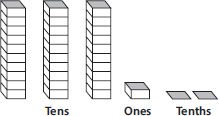(B)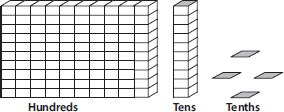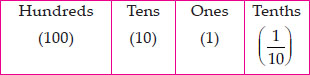SOLUTION: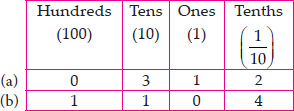Q 2.

Write the following decimals in the place value table.
(A) 19.4
(B) 0.3
(C) 10.6
(D) 205.9

SOLUTION:

(A)

 Hundreds Tens Ones Tenths 0 1 9 4

(B)
 Hundreds Tens Ones Tenths 0 0 0 3

(C)
 Hundreds Tens Ones Tenths 0 1 0 6

(D)
 Hundreds Tens Ones Tenths 2 0 5 9

Q 3.

Write each of the following as decimals :
(A) Seven-tenths
(B) Two tens and nine-tenths
(C) Fourteen point six
(D) One hundred and two ones
(E) Six hundred point eight

SOLUTION:

(A) seven-tenths = 7 tenths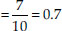(B) Two tens and nine-tenths = 2 × 10 +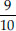= 20 + 0.9 = 20.9
(C) Fourteen point six = 14.6
(D) One hundred and two-ones = 100 + 2 × 1
= 100 + 2 = 102.0
(E) Six hundred point eight = 600.8

Q 4.

Write each of the following as decimals:
(A)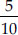(B)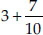(C) 200 + 60 + 5 +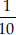(D)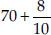(E)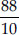(F)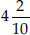(G)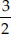(H)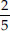(I)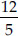(J)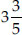(K)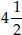SOLUTION: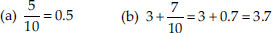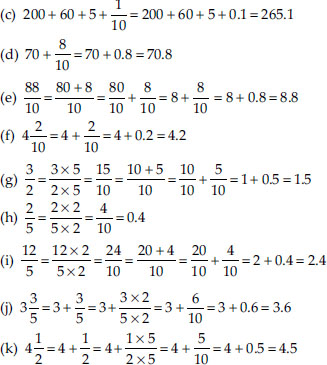Q 5.

Write the following decimals as fractions. Reduce the fractions to lowest form.
(A) 0.6
(B) 2.5
(C) 1.0
(D) 3.8
(E) 13.7
(F) 21.2
(G) 6.4

SOLUTION: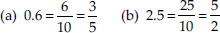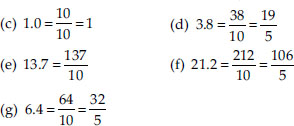Q 6.

Express the following as cm using decimals.
(A) 2 mm
(B) 30 mm
(C) 116 mm
(D) 4 cm 2 mm
(E) 162 mm
(F) 83 mm

SOLUTION:

(A) ∴ 10 mm = 1 cm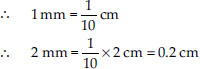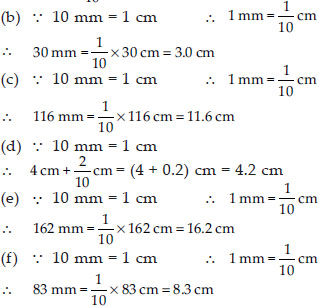Q 7.

Between which two whole numbers on the number line are the given numbers lie?
Which of these whole numbers is nearer the number?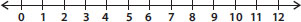(A) 0.8
(B) 5.1
(C) 2.6
(D) 6.4
(E) 9.1
(F) 4.9

SOLUTION:

(A) 0.8 lies between 0 and 1. From 0 to 1, 0.8 is nearer to 1.
(B) 5.1 lies between 5 and 6. From 5 to 6, 5.1 is nearer to 5.
(C) 2.6 lies between 2 and 3. From 2 to 3, 2.6 is nearer to 3.
(D) 6.4 lies between 6 and 7. from 6 to 7, 6.4 is nearer to 6.
(E) 9.1 lies between 9 and 10. From 9 to 10, 9.1 is nearer to 9.
(F) 4.9 lies between 4 and 5. From 4 to 5, 4.9 is nearer to 5.

Q 8.

Show the following numbers on the number line.
(A) 0.2
(B) 1.9
(C) 1.1
(D) 2.5

SOLUTION: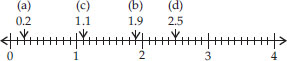Q 9.

Write the decimal number represented by the points A, B, C, D on the given number line.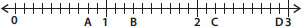SOLUTION: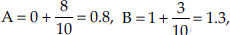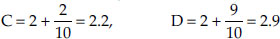Q 10.

(A) The length of Ramesh's notebook is 9 cm 5 mm. What will be its length in cm?
(B) The length of a young gram plant is 65 mm. Express its length in cm.

SOLUTION:

(A) 9 cm 5 mm = 9 cm + 5 mm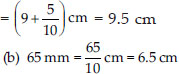Q 11.

Complete the table with the help of these boxes and use decimals to write the number.
(A)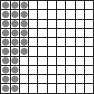(B)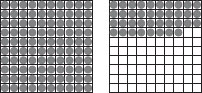(C)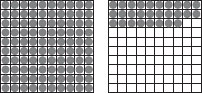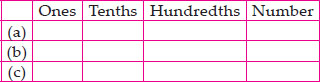SOLUTION: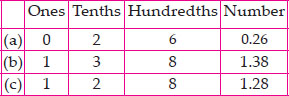Q 12.

Write the numbers given in the following place value table in decimal form.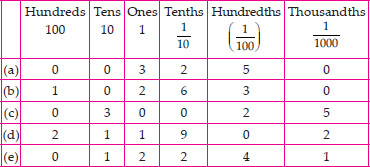SOLUTION:

(A) 0 × 100 + 0 × 10 + 3 × 1 +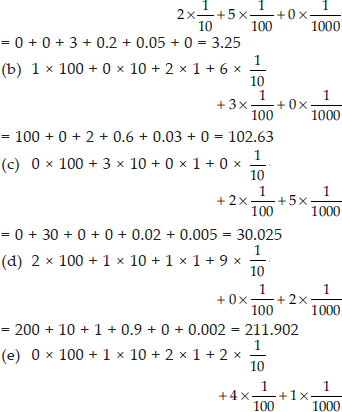= 0 + 10 + 2 + 0.2 + 0.04 + 0.001 = 12.241

Q 13.

Write the following decimals in the place value table.
(A) 0.29
(B) 2.08
(C) 19.60
(D) 148.32
(E) 200.812

SOLUTION: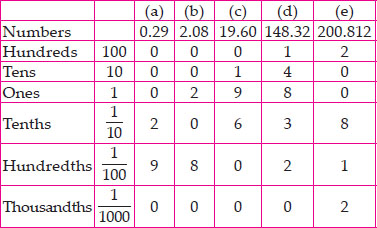Q 14.

Write each of the following as decimals.
(A)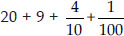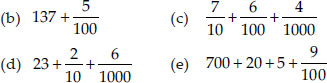SOLUTION:

(A) 20 + 9 + 0.4 + 0.01 = 29.41
(B) 137 + 0.05 = 137.05
(C) 0.7 + 0.06 + 0.004 = 0.764
(D) 23 + 0.2 + 0.006 = 23.206
(E) 700 + 20 + 5 + 0.09 = 725.09

Q 15.

Write each of the following decimals in words.
(A) 0.03
(B) 1.20
(C) 108.56
(D) 10.07
(E) 0.032
(F) 5.008

SOLUTION:

(A) Zero point zero three
(B) One point two zero
(C) One hundred eight point five six
(D) Ten point zero seven
(E) Zero point zero three two
(F) Five point zero zero eight

Q 16.

Between which two numbers in tenths place on the number line does each of the given number lie?
(A) 0.06
(B) 0.45
(C) 0.19
(D) 0.66
(E) 0.92
(F) 0.57

SOLUTION:

(A) 0.06 lies between 0 and 0.1.
(B) 0.45 lies between 0.4 and 0.5.
(C) 0.19 lies between 0.1 and 0.2.
(D) 0.66 lies between 0.6 and 0.7.
(E) 0.92 lies between 0.9 and 1.0.
(F) 0.57 lies between 0.5 and 0.6.

Q 17.

Write as fractions in lowest terms.
(A) 0.60
(B) 0.05
(C) 0.75
(D) 0.18
(E) 0.25
(F) 0.125
(G) 0.066

SOLUTION: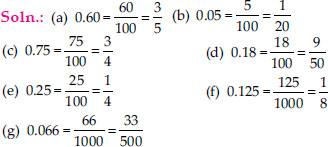Q 18.

Which is greater?
(A) 0.3 or 0.4
(B) 0.07 or 0.02
(C) 3 or 0.8
(D) 0.5 or 0.05
(E) 1.23 or 1.2
(F) 0.099 or 0.19
(G) 1.5 or 1.50
(H) 1.431 or 1.490
(I) 3.3 or 3.300
(J) 5.64 or 5.603

SOLUTION:

Before comparing, we write both terms in like decimals :
(A) 0.3 < 0.4
(B) 0.07 > 0.02
(C) 3.0 or 0.8 ⇒ 3.0 > 0.8
(D) 0.50 or 0.05 ⇒ 0.50 > 0.05
(E) 1.23 or 1.20 ⇒ 1.23 > 1.20
(F) 0.099 or 0.190 ⇒ 0.099 < 0.190
(G) 1.50 or 1.50 ⇒ 1.50 = 1.50
(H) 1.431 < 1.490
(I) 3.300 or 3.300 ⇒ 3.300 = 3.300
(J) 5.640 or 5.603 ⇒ 5.640 > 5.603

Q 19.

Make five more examples and find the greater number from them.

SOLUTION:

Five examples are :
(I) 1.8 or 1.82
(ii) 1.0009 or 1.09
(iii) 10.01 or 100.1
(iv) 5.1 or 5.01
(v) 4.213 or 421.3
Before comparing, we write both terms in like decimals
(I) 1.80 or 1.82 ⇒ 1.82 is greater than 1.8
(ii) 1.0009 or 1.0900 ⇒ 1.09 is greater than 1.0009
(iii) 10.01 or 100.10 ⇒ 100.1 is greater than 10.01
(iv) 5.10 or 5.01 ⇒ 5.1 is greater than 5.01
(v) 4.213 or 421.300 ⇒ 421.3 is greater than 4.213

Q 20.

Express as rupees using decimals.
(A) 5 paise
(B) 75 paise
(C) 20 paise
(D) 50 rupees 90 paise
(E) 725 paise

SOLUTION: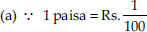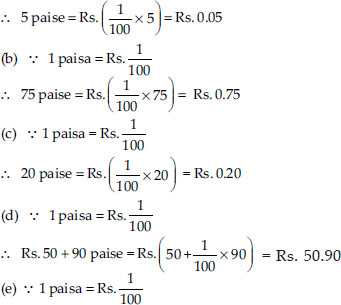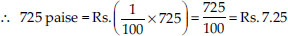Q 21.

Express as metres using decimals.
(A) 15 cm
(B) 6 cm
(C) 2 m 45 cm
(D) 9 m 7 cm
(E) 419 cm

SOLUTION: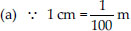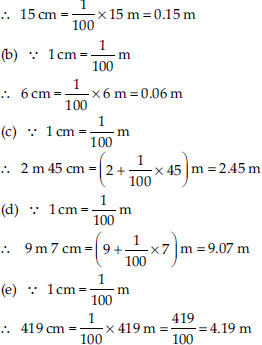Q 22.

Express as cm using decimals.
(A) 5 mm
(B) 60 mm
(C) 164 mm
(D) 9 cm 8 mm
(E) 93 mm

SOLUTION: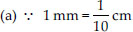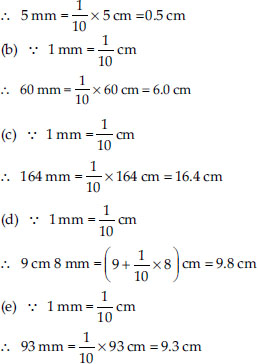Q 23.

Express as km using decimals.
(A) 8 m
(B) 88 m
(C) 8888 m
(D) 70 km 5 m

SOLUTION: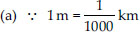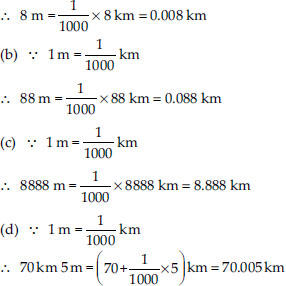Q 24.

Express as kg using decimals.
(A) 2 g
(B) 100 g
(C) 3750 g
(D) 5 kg 8 g
(E) 26 kg 50 g

SOLUTION: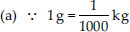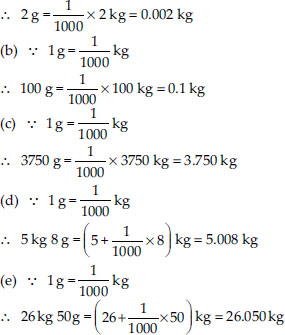Q 25.

Find the sum in each of the following :
(A) 0.007 + 8.5 + 30.08
(B) 15 + 0.632 + 13.8
(C) 27.076 + 0.55 + 0.004
(D) 25.65 + 9.005 + 3.7
(E) 0.75 + 10.425 + 2
(F) 280.69 + 25.2 + 38

SOLUTION:

(A)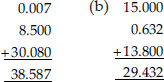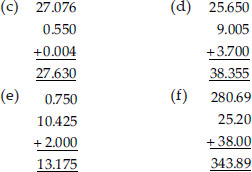Q 26.

Rashid spent Rs. 35.75 for Maths book and Rs. 32.60 for Science book. Find the total amount spent by Rashid.

SOLUTION:

Money spent for Maths book = Rs. 35.75
Money spent for Science book = Rs. 32.60
Total money spent = Rs. 35.75 + Rs. 32.60
= Rs. 68.35
Therefore, total money spent by Rashid is Rs. 68.35.

Q 27.

Radhika's mother gave her Rs. 10.50 and her father gave her Rs. 15.80, find the total amount given to Radhika by the parents.

SOLUTION:

Money given by mother = Rs. 10.50
Money given by father = Rs. 15.80
= Rs. 10.50 + Rs. 15.80 = Rs. 26.30

Q 28.

Nasreen bought 3 m 20 cm cloth for her shirt and 2 m 5 cm cloth for her trouser. Find the total length of cloth bought by her.

SOLUTION:

Cloth bought for shirt = 3 m 20 cm
= 3.20 m
Cloth bought for trouser = 2 m 5 cm = 2.05 m
Total length of cloth bought by Nasreen
= 3.20 m + 2.05 m = 5.25 m
Therefore, total length of cloth bought by Nasreen is 5.25 m.

Q 29.

Naresh walked 2 km 35 m in the morning and 1 km 7 m in the evening. How much distance did he walk in all?

SOLUTION:

Distance travelled in the morning
= 2 km 35 m = 2.035 km
Distance travelled in the evening
= 1 km 7 m = 1.007 km
Total distance travelled
= (2.035 + 1.007) km = 3.042 km
Therefore, total distance travelled by Naresh is 3.042 km.

Q 30.

Sunita travelled 15 km 268 m by bus, 7 km 7 m by car and 500 m on foot in order to reach her school. How far is her school from her residence?

SOLUTION:

Distance travelled by bus = 15 km 268 m
= 15.268 km
Distance travelled by car = 7 km 7 m
= 7.007 km
Distance travelled on foot = 500 m
= 0.500 km
Total distance travelled
= (15.268 + 7.007 + 0.500) km = 22.775 km
Therefore, total distance travelled by Sunita is 22.775 km.

Q 31.

Ravi purchased 5 kg 400 g rice, 2 kg 20 g sugar and 10 kg 850g flour. Find the total weight of his purchases.

SOLUTION:

Weight of rice = 5 kg 400 g = 5.400 kg
Weight of sugar = 2 kg 20 g = 2.020 kg
Weight of flour = 10 kg 850 g = 10.850 kg
Total weight = (5.400 + 2.020 + 10.850) kg
= 18.270 kg
Therefore, total weight of Ravi's purchases is 18.270 kg.

Q 32.

Subtract :
(A) Rs. 18.25 from Rs. 20.75
(B) 202.54 m from 250 m
(C) Rs. 5.36 from Rs. 8.40
(D) 2.051 km from 5.206 km
(E) 0.314 kg from 2.107 kg

SOLUTION:

(A)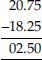∴ Rs. 20.75 - Rs. 18.25 = Rs. 2.50
(B)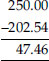∴ 250 m - 202.54 m = 47.46 m
(C)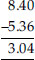∴ Rs. 8.40 - Rs. 5.36 = Rs. 3.04
(D)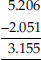∴ 5.206 km - 2.051 km = 3.155 km
(E)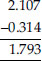∴ 2.107 kg - 0.314 kg = 1.793 kg

Q 33.

Find the value of :
(A) 9.756 - 6.28
(B) 21.05 - 15.27
(C) 18.5 - 6.79
(D) 11.6 - 9.847

SOLUTION:

(A)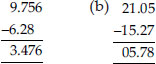(C)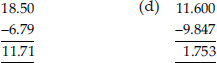Q 34.

Raju bought a book for Rs. 35.65. He gave Rs. 50 to the shopkeeper. How much money did he get back from the shopkeeper?

SOLUTION:

Total amount given to shopkeeper = Rs. 50
Cost of book = Rs. 35.65
Amount left = Rs. 50.00 - Rs. 35.65 = Rs. 14.35
Therefore, Raju got back Rs. 14.35 from the shopkeeper.

Q 35.

Rani had Rs. 18.50. She bought one ice-cream for Rs. 11.75. How much money does she have now?

SOLUTION:

Total money = Rs. 18.50
Cost of ice-cream = Rs. 11.75
Amount left = Rs. 18.50 - Rs. 11.75 = Rs. 6.75
Therefore, Rani has Rs. 6.75 now.

Q 36.

Tina had 20 m 5 cm long cloth. She cuts 4 m 50 cm length of cloth from this for making a curtain. How much cloth is left with her?

SOLUTION:

Total length of cloth = 20 m 5 cm
= 20.05 m
Length of cloth used = 4 m 50 cm = 4.50 m
Remaining cloth = 20.05 m - 4.50 m = 15.55 m
Therefore, 15.55 m of cloth is left with Tina.

Q 37.

Namita travels 20 km 50 m every day. Out of this she travels 10 km 200 m by bus and the rest by auto. How much distance does she travel by auto?

SOLUTION:

Total distance travelled = 20 km 50 m
= 20.050 km
Distance travelled by bus = 10 km 200 m
= 10.200 km
Distance travelled by auto
= (20.050 - 10.200) km = 9.850 km
Therefore, 9.850 km distance is travelled by auto.

Q 38.

Aakash bought vegetables weighing 10 kg. Out of this, 3 kg 500 g is onions, 2 kg 75 g is tomatoes and the rest is potatoes. What is the weight of the potatoes?

SOLUTION:

Weight of onions = 3 kg 500 g = 3.500 kg
Weight of tomatoes = 2 kg 75 g = 2.075 kg
Total weight of onions and tomatoes
= (3.500 + 2.075) kg = 5.575 kg
Therefore, weight of potatoes
= (10.000 - 5.575) kg = 4.425 kg
Thus, weight of potatoes is 4.425 kg.